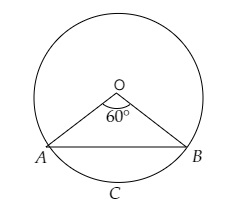# A chord $A B$ of a circle, of radius $14 \mathrm{~cm}$ makes an angle of $60^{\circ}$ at the centre of the circle. Find the area of the minor segment of the circle. (Use $\pi=22 / 7)$

Given:

A chord $A B$ of a circle, of radius $14 \mathrm{~cm}$ makes an angle of $60^{\circ}$ at the centre of the circle.

To do:

We have to find the area of the minor segment of the circle.

Solution:

Radius of the circle $r = 14\ cm$
Angle subtended at the centre $\angle A0B = 60^o$Area of the minor segment $\mathrm{ACB}=(\frac{\theta \pi}{360^{\circ}}-\sin \frac{\theta}{2} \cos \frac{\theta}{2}) r^2$

$=(\frac{60^{\circ} \times 22}{360^{\circ} \times 7} - sin \frac{60^{\circ}}{2} \cos \frac{60^{\circ}}{2})(14)^{2}$

$=196(\frac{11}{21}-\sin 30^{\circ} \cos 30^{\circ})$

$=196(\frac{11}{21}-\frac{1}{2} \times \frac{\sqrt{3}}{2})$

$=196(\frac{44-21 \sqrt{3}}{84})$

$=\frac{196}{84}(44-21 \times 1.732)$

$=\frac{7}{3}(44-36.37)$

$=\frac{7}{3}(7.63)$

$=17.80 \mathrm{~cm}^{2}$

The area of the minor segment of the circle is $17.80\ cm^2$.

Updated on: 10-Oct-2022

2K+ Views